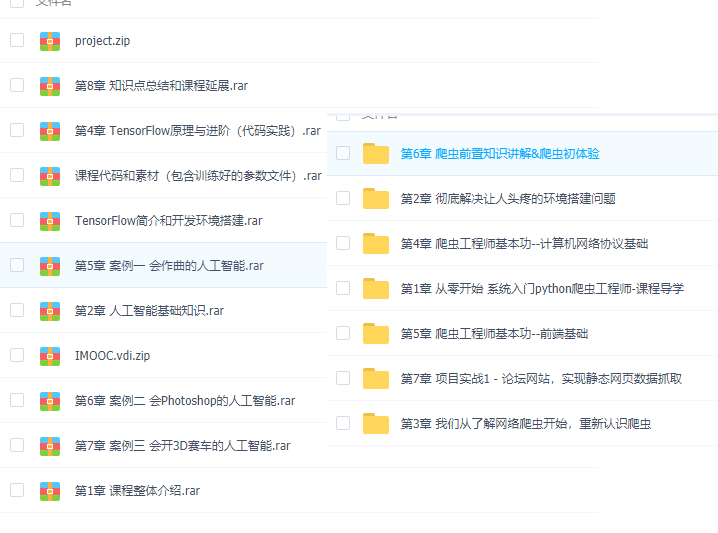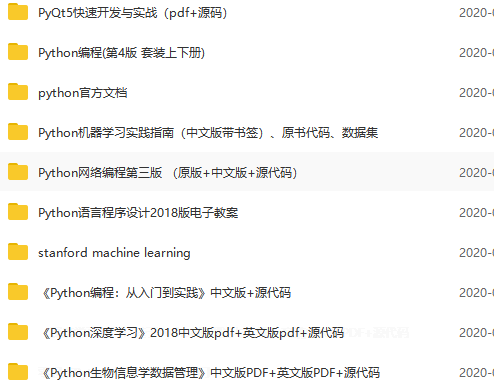# 建议你吃透python这68个内置函数！

abs()           dict()        help()         min()         setattr()
all()           dir()         hex()          next()        slice()
any()           divmod()      id()           object()      sorted()
ascii()         enumerate()   input()        oct()         staticmethod()
bin()           eval()        int()          open()        str()
bool()          exec()        isinstance()   ord()         sum()
bytearray()     ﬁlter()       issubclass()   pow()         super()
bytes()         ﬂoat()        iter()         print()       tuple()
callable()      format()      len()          property()    type()
chr()           frozenset()   list()         range()       vars()
classmethod()   getattr()     locals()       repr()        zip()
compile()       globals()     map()          reversed()    __import__()
complex()       hasattr()     max()          round()
delattr()       hash()        memoryview()   set()


• 和数字相关
• 1. 数据类型
• 2. 进制转换
• 3. 数学运算
• 和数据结构相关
• 1. 序列
• 2. 数据集合
• 3. 相关内置函数
• 和作用域相关
• 和迭代器生成器相关
• 字符串类型代码的执行
• 输入输出
• 内存相关
• 文件操作相关
• 模块相关
• 帮 助
• 调用相关
• 查看内置属性

## 和数字相关

### 1. 数据类型

• bool : 布尔型(True,False)
• int : 整型(整数)
• float : 浮点型(小数)
• complex : 复数

### 2. 进制转换

• bin() 将给的参数转换成二进制
• otc() 将给的参数转换成八进制
• hex() 将给的参数转换成十六进制
print(bin(10))  # 二进制:0b1010
print(hex(10))  # 十六进制:0xa
print(oct(10))  # 八进制:0o12


### 3. 数学运算

• abs() 返回绝对值
• divmode() 返回商和余数
• round() 四舍五入
• pow(a, b) 求a的b次幂, 如果有三个参数. 则求完次幂后对第三个数取余
• sum() 求和
• min() 求最小值
• max() 求最大值
print(abs(-2))  # 绝对值:2
print(divmod(20,3)) # 求商和余数:(6,2)
print(round(4.50))   # 五舍六入:4
print(round(4.51))   #5
print(pow(10,2,3))  # 如果给了第三个参数. 表示最后取余:1
print(sum([1,2,3,4,5,6,7,8,9,10]))  # 求和:55
print(min(5,3,9,12,7,2))  #求最小值:2
print(max(7,3,15,9,4,13))  #求最大值:15


## 和数据结构相关

### 1. 序列

（1）列表和元组

• list() 将一个可迭代对象转换成列表
• tuple() 将一个可迭代对象转换成元组
print(list((1,2,3,4,5,6)))  #[1, 2, 3, 4, 5, 6]
print(tuple([1,2,3,4,5,6]))  #(1, 2, 3, 4, 5, 6)


（2）相关内置函数

• reversed() 将一个序列翻转, 返回翻转序列的迭代器
• slice() 列表的切片
lst = "你好啊"
it = reversed(lst)   # 不会改变原列表. 返回一个迭代器, 设计上的一个规则
print(list(it))  #['啊', '好', '你']
lst = [1, 2, 3, 4, 5, 6, 7]
print(lst[1:3:1])  #[2,3]
s = slice(1, 3, 1)  #  切片用的
print(lst[s])  #[2,3]


（3）字符串

• str() 将数据转化成字符串
print(str(123)+'456')  #123456


• format() 与具体数据相关, 用于计算各种小数, 精算等.
s = "hello world!"
print(format(s, "^20"))  #剧中
print(format(s, "<20"))  #左对齐
print(format(s, ">20"))  #右对齐
#     hello world!
# hello world!
#         hello world!
print(format(3, 'b' ))    # 二进制:11
print(format(97, 'c' ))   # 转换成unicode字符:a
print(format(11, 'd' ))   # ⼗进制:11
print(format(11, 'o' ))   # 八进制:13
print(format(11, 'x' ))   # 十六进制(⼩写字母):b
print(format(11, 'X' ))   # 十六进制(大写字母):B
print(format(11, 'n' ))   # 和d⼀样:11
print(format(11))         # 和d⼀样:11
print(format(123456789, 'e' ))      # 科学计数法. 默认保留6位小数:1.234568e+08
print(format(123456789, '0.2e' ))   # 科学计数法. 保留2位小数(小写):1.23e+08
print(format(123456789, '0.2E' ))   # 科学计数法. 保留2位小数(大写):1.23E+08
print(format(1.23456789, 'f' ))     # 小数点计数法. 保留6位小数:1.234568
print(format(1.23456789, '0.2f' ))  # 小数点计数法. 保留2位小数:1.23
print(format(1.23456789, '0.10f'))  # 小数点计数法. 保留10位小数:1.2345678900
print(format(1.23456789e+3, 'F'))   # 小数点计数法. 很大的时候输出INF:1234.567890


• bytes() 把字符串转化成bytes类型
bs = bytes("今天吃饭了吗", encoding="utf-8")


• bytearray() 返回一个新字节数组. 这个数字的元素是可变的, 并且每个元素的值得范围是[0,256)
ret = bytearray("alex" ,encoding ='utf-8')
print(ret)  #97
print(ret)  #bytearray(b'alex')
ret = 65  #把65的位置A赋值给ret
print(str(ret))  #bytearray(b'Alex')


• ord() 输入字符找带字符编码的位置
• chr() 输入位置数字找出对应的字符
• ascii() 是ascii码中的返回该值 不是就返回u
print(ord('a'))  # 字母a在编码表中的码位:97
print(ord('中'))  # '中'字在编码表中的位置:20013
print(chr(65))  # 已知码位,求字符是什么:A
print(chr(19999))  #丟

for i in range(65536):  #打印出0到65535的字符
print(chr(i), end=" ")

print(ascii("@"))  #'@'


• repr() 返回一个对象的string形式
s = "今天\n吃了%s顿\t饭" % 3
print(s)#今天# 吃了3顿    饭
print(repr(s))   # 原样输出,过滤掉转义字符 \n \t \r 不管百分号%
#'今天\n吃了3顿\t饭'


### 2. 数据集合

• 字典：dict 创建一个字典
• 集合：set 创建一个集合

frozenset() 创建一个冻结的集合，冻结的集合不能进行添加和删除操作。

### 3. 相关内置函数

• len() 返回一个对象中的元素的个数
• sorted() 对可迭代对象进行排序操作 (lamda)

• Iterable: 可迭代对象
• key: 排序规则(排序函数), 在sorted内部会将可迭代对象中的每一个元素传递给这个函数的参数. 根据函数运算的结果进行排序
• reverse: 是否是倒叙. True: 倒叙, False: 正序
lst = [5,7,6,12,1,13,9,18,5]
lst.sort()  # sort是list里面的一个方法
print(lst)  #[1, 5, 5, 6, 7, 9, 12, 13, 18]

ll = sorted(lst) # 内置函数. 返回给你一个新列表  新列表是被排序的
print(ll)  #[1, 5, 5, 6, 7, 9, 12, 13, 18]

l2 = sorted(lst,reverse=True)  #倒序
print(l2)  #[18, 13, 12, 9, 7, 6, 5, 5, 1]

#根据字符串长度给列表排序
lst = ['one', 'two', 'three', 'four', 'five', 'six']
def f(s):
return len(s)
l1 = sorted(lst, key=f, )
print(l1)  #['one', 'two', 'six', 'four', 'five', 'three']


• enumerate() 获取集合的枚举对象
lst = ['one','two','three','four','five']
for index, el in enumerate(lst,1):    # 把索引和元素一起获取,索引默认从0开始. 可以更改
print(index)
print(el)
# 1
# one
# 2
# two
# 3
# three
# 4
# four
# 5
# five


• all() 可迭代对象中全部是True, 结果才是True
• any() 可迭代对象中有一个是True, 结果就是True
print(all([1,'hello',True,9]))  #True
print(any([0,0,0,False,1,'good']))  #True

• zip() 函数用于将可迭代的对象作为参数, 将对象中对应的元素打包成一个元组, 然后返回由这些元组组成的列表. 如果各个迭代器的元素个数不一致, 则返回列表长度与最短的对象相同
lst1 = [1, 2, 3, 4, 5, 6]
lst2 = ['醉乡民谣', '驴得水', '放牛班的春天', '美丽人生', '辩护人', '被嫌弃的松子的一生']
lst3 = ['美国', '中国', '法国', '意大利', '韩国', '日本']
print(zip(lst1, lst1, lst3))  #<zip object at 0x00000256CA6C7A88>
for el in zip(lst1, lst2, lst3):
print(el)
# (1, '醉乡民谣', '美国')
# (2, '驴得水', '中国')
# (3, '放牛班的春天', '法国')
# (4, '美丽人生', '意大利')
# (5, '辩护人', '韩国')
# (6, '被嫌弃的松子的一生', '日本')


• fiter() 过滤 (lamda)

function: 用来筛选的函数. 在ﬁlter中会自动的把iterable中的元素传递给function. 然后根据function返回的True或者False来判断是否保留留此项数据 , Iterable: 可迭代对象

def func(i):    # 判断奇数
return i % 2 == 1
lst = [1,2,3,4,5,6,7,8,9]
l1 = filter(func, lst)  #l1是迭代器
print(l1)  #<filter object at 0x000001CE3CA98AC8>
print(list(l1))  #[1, 3, 5, 7, 9]


• map() 会根据提供的函数对指定序列列做映射(lamda)

def f(i):
return i
lst = [1,2,3,4,5,6,7,]
it = map(f, lst) # 把可迭代对象中的每一个元素传递给前面的函数进行处理. 处理的结果会返回成迭代器print(list(it))  #[1, 2, 3, 4, 5, 6, 7]


## 和作用域相关

• locals() 返回当前作用域中的名字
• globals() 返回全局作用域中的名字
def func():
a = 10
print(locals())  # 当前作用域中的内容
print(globals())  # 全局作用域中的内容
print("今天内容很多")
func()
# {'a': 10}
# {'__name__': '__main__', '__doc__': None, '__package__': None, '__loader__':
# '__spec__': None, '__annotations__': {}, '__builtins__': <module 'builtins'
# (built-in)>, '__file__': 'D:/pycharm/练习/week03/new14.py', '__cached__': None,
#  'func': <function func at 0x0000026F8D6B97B8>}
# 今天内容很多


## 和迭代器生成器相关

• range() 生成数据
• next() 迭代器向下执行一次, 内部实际使用了__ next__()方法返回迭代器的下一个项目
• iter() 获取迭代器, 内部实际使用的是__ iter__()方法来获取迭代器
for i in range(15,-1,-5):
print(i)
# 15
# 10
# 5
# 0
lst = [1,2,3,4,5]
it = iter(lst)  #  __iter__()获得迭代器
print(it.__next__())  #1
print(next(it)) #2  __next__()
print(next(it))  #3
print(next(it))  #4


## 字符串类型代码的执行

• eval() 执行字符串类型的代码. 并返回最终结果
• exec() 执行字符串类型的代码
• compile() 将字符串类型的代码编码. 代码对象能够通过exec语句来执行或者eval()进行求值
s1 = input("请输入a+b:")  #输入:8+9
print(eval(s1))  # 17 可以动态的执行代码. 代码必须有返回值
s2 = "for i in range(5): print(i)"
a = exec(s2) # exec 执行代码不返回任何内容

# 0
# 1
# 2
# 3
# 4
print(a)  #None

# 动态执行代码
exec("""
def func():
print(" 我是周杰伦")
""" )
func()  #我是周杰伦

code1 = "for i in range(3): print(i)"
com = compile(code1, "", mode="exec")   # compile并不会执行你的代码.只是编译
exec(com)   # 执行编译的结果
# 0
# 1
# 2

code2 = "5+6+7"
com2 = compile(code2, "", mode="eval")
print(eval(com2))  # 18

code3 = "name = input('请输入你的名字:')"  #输入:hello
com3 = compile(code3, "", mode="single")
exec(com3)
print(name)  #hello


## 输入输出

• print() : 打印输出
• input() : 获取用户输出的内容
print("hello", "world", sep="*", end="@") # sep:打印出的内容用什么连接,end:以什么为结尾
#hello*world@


## 内存相关

• hash() : 获取到对象的哈希值(int, str, bool, tuple). hash算法:(1) 目的是唯一性 (2) dict 查找效率非常高, hash表.用空间换的时间 比较耗费内存
s = 'alex'
print(hash(s))  #-168324845050430382
lst = [1, 2, 3, 4, 5]
print(hash(lst))  #报错,列表是不可哈希的
id() :  获取到对象的内存地址
s = 'alex'
print(id(s))  #2278345368944


文件操作相关
• open() : 用于打开一个文件, 创建一个文件句柄
f = open('file',mode='r',encoding='utf-8')
f.close()


## 模块相关

__ import__() : 用于动态加载类和函数

# 让用户输入一个要导入的模块
import os
name = input("请输入你要导入的模块:")
__import__(name)    # 可以动态导入模块


## 帮 助

• help() : 函数用于查看函数或模块用途的详细说明
print(help(str))  #查看字符串的用途


## 调用相关

• callable() : 用于检查一个对象是否是可调用的. 如果返回True, object有可能调用失败, 但如果返回False. 那调用绝对不会成功
a = 10
print(callable(a))  #False  变量a不能被调用
#
def f():
print("hello")
print(callable(f))   # True 函数是可以被调用的

## 查看内置属性

• dir() : 查看对象的内置属性, 访问的是对象中的__dir__()方法
print(dir(tuple))  #查看元组的方法## 最新python项目教程，敬请关注 我的专栏python

posted @ 2020-10-22 21:05  八重樱  阅读(476)  评论(0编辑  收藏  举报